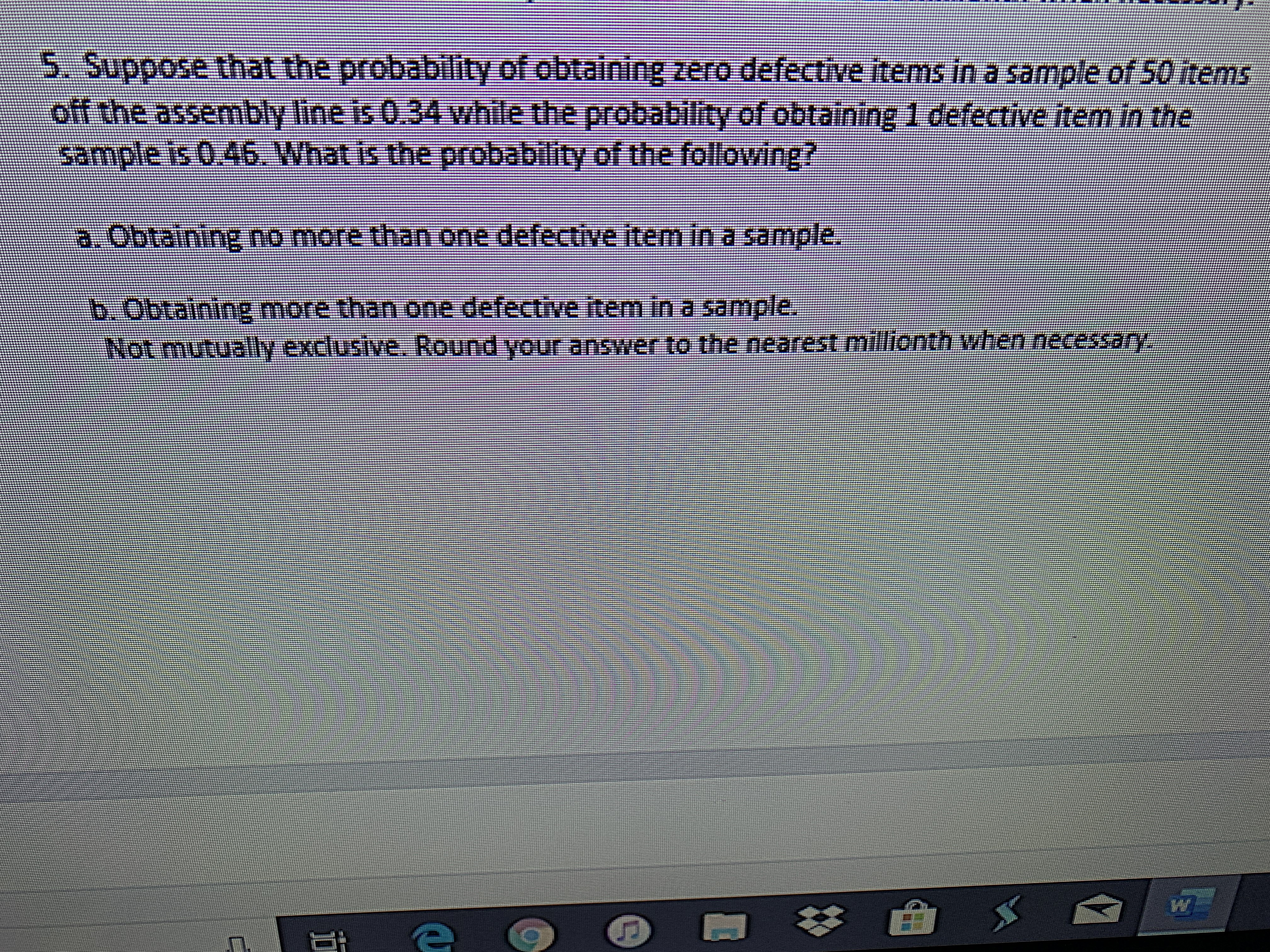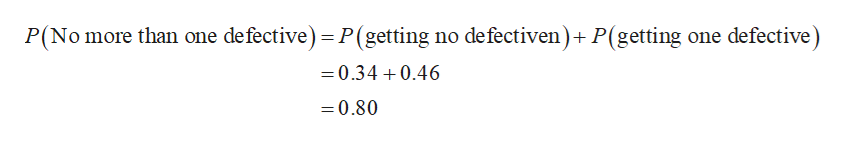# 5. Suppose that the probability of obtaining zero defective items in a sample of 50 itemsoff the assembly line is 0.34 while the probability of obtaining 1 defective item in thesample is 0:46. What is the probability of the following?a.Obtaining no more than one defective item in a sample.b.Obtaining more than one defective item in a sarmple.Not mutualy exclusive. Round your answer to the nearest millionth when necessany

Question
56 viewshelp_outlineImage Transcriptionclose5. Suppose that the probability of obtaining zero defective items in a sample of 50 items off the assembly line is 0.34 while the probability of obtaining 1 defective item in the sample is 0:46. What is the probability of the following? a.Obtaining no more than one defective item in a sample. b.Obtaining more than one defective item in a sarmple. Not mutualy exclusive. Round your answer to the nearest millionth when necessany fullscreen
check_circle

Step 1

It is given that P(getting zero defective items) is 0.34 and P(getting 1 defective items) is 0.46.

Step 2

a)

The required probability is ...help_outlineImage TranscriptioncloseP(No more than one defective) P(getting no defectiven)+ P(getting one defective) =0.34 0.46 =0.80 fullscreen

### Want to see the full answer?

See Solution

#### Want to see this answer and more?

Solutions are written by subject experts who are available 24/7. Questions are typically answered within 1 hour.*

See Solution
*Response times may vary by subject and question.
Tagged in

### Statistics